# Clustering analysis : Key concepts and Implementation in Python

hyangki0119·2022년 3월 28일
0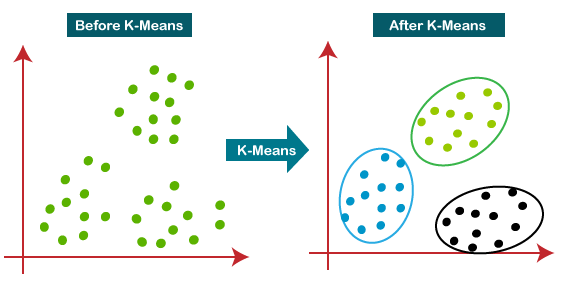# Clustering : Introduction

## What is clustering?

To partition the observations into groups($clusters$) so that the pairwise dissimilarities between those assigned to the same cluster tend to be smaller than those in differenct clusters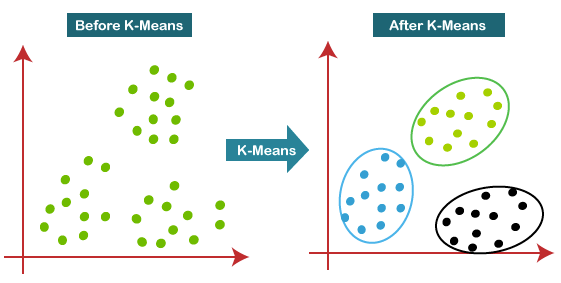## Types of clustering algorithm

TypeDescriptionExample
Combinatorial
algorithms
Work directly on the observed data with no direct reference to an underlyning probability model
Iterative descent
clustering
K-means clustering
Mixture
modeling
Supposes that the data is an i.i.d sample from some population described by a probability distribution functionMixture model
Mode
seekers
Take a nonparametric perspective, attempting to directly estimate distinct mode of the probability distribution functionPatient Rule Induction Method(PRIM),
Generalized Association Rules
Hierachical
clustering

## Iterative greedy descent clustering

• An initial partition is specified
• At each iterative step, the cluster assignment are changed in such a way that the value of the criterion is improved from its previous value
• When the prescription is unable to provide an improvement, the algorithm terminates with the current assignmnet as its solution

# Example data generation

• Make a toy data using make_blobs in sklearn!
from sklearn.datasets import make_blobs

x, y = make_blobs(n_samples=100, centers=3, n_features=2, random_state=7)
points = pd.DataFrame(x, y).reset_index(drop=True)
points.columns = ["x", "y"]
points.head()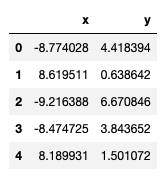import seaborn as sns
sns.scatterplot(x="x", y="y", data=points, palette="Set2");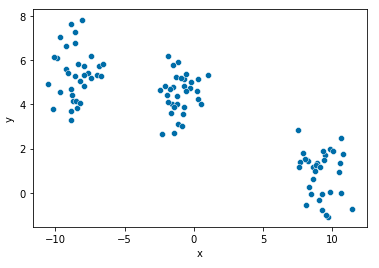# 1. K-means

• The K-means algorithms is one of the most popular iterative descent clustering methods

## Algorithm

GivenChange
Step1Cluster assignmentChange the means of cluster
Step2A current set of meansAssigning of each observations
1. For a given cluster assignment, the total variance is minimized with respect to $\{m_{1},\dots,m_{K}\}$ yielding the means of the currently assigned cluster
$\bar{x}_{S} = \argmin_{m} \sum_{i\in S} \lvert\lvert x_i - m \rvert\rvert^{2}$
1. Given a current set of means $\{m_{1},\dots,m_{K}\}$, the total variance is minimized by assigning each observation to the closest (current) cluster mean
$C(i) = \argmin_{1\le k\le K} \lvert\lvert x_i - m_k \rvert\rvert^{2}$
1. Step 1 and 2 are iterated until the assignmnets do not change

## Notice on intial centroids

• One should start the algorithm with many different random choices for the starting means, abd choose the solution having smallest value of the objective fucntion

# 2. Gaussian mixture

## EM algorithm

StepGivenUpdate
E stepMixture component parametersResponsibilities
M stepResponsibilitiesMixture component parameters
• E step
• Assigns responsibilities for each data point based in its relative density under each mixture component
• M step
• Recomputes the component density parameters based on the current responsibilities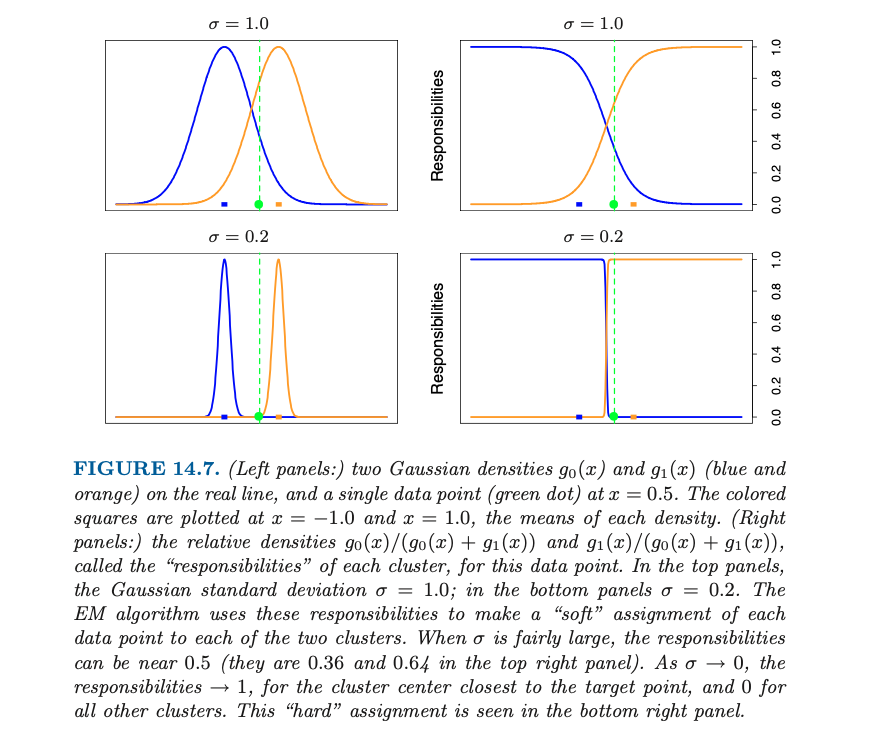• Responsibilities
• For given two density $g_0$ and $g_1$ and a data point $x$, the relative densities
$\frac{g_0(x)}{g_0(x) + g_1(x)}, \quad\frac{g_1(x)}{g_0(x) + g_1(x)}$
are called the $responsibility$ of each cluster, for this data point $x$

## Gaussian mixture as soft K-means clustering

• EM algorithm is a soft version of K-means clustering, making probabilistic (rather than deterministic) assignments of points to cluster centers
• As $\sigma^2\to 0$, these probabilities become 0 and 1, and the two methods K-means and Gaussian mixture coincide

# 3. Hierachical clustering

## Gap statistics

Tibishirani proposed a statistic for choosing optimal number of clusters in 2001, called $gap\text{ }statistics$: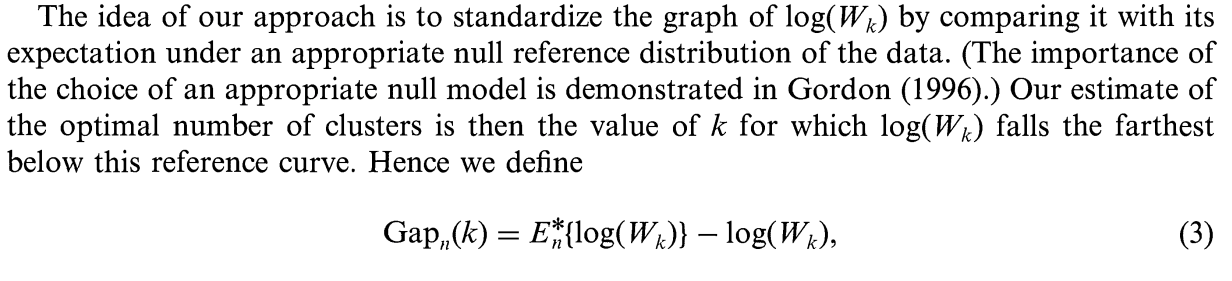## Dendrogram

A $dendrogram$ provides a highly interpretable complete description of the hierachical clustering in a graphical format.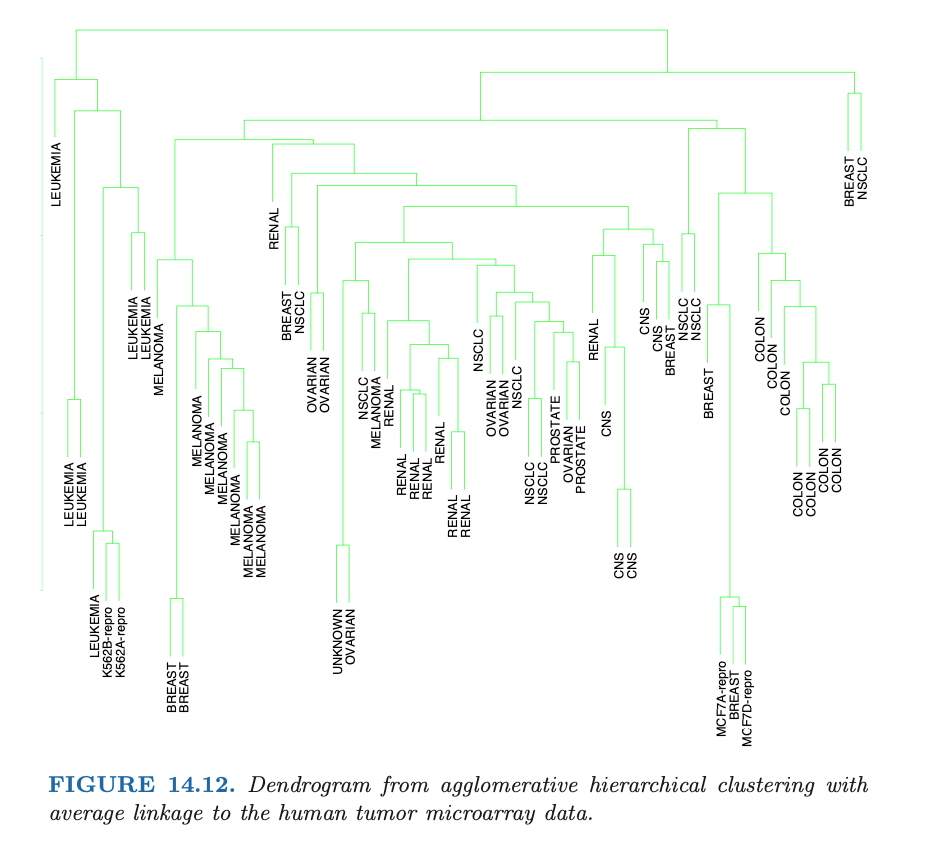## Agglomerative vs. Divisive

AgglomerativeDivisive
Bottom upTop down
Recursively mergeRecursively split
Begin with every observation representing a singleton clusterBegin with the entire data set as a single cluster
• Agglomerative clustering
• Begin with every observation representing a singleton cluster
• At each of the $N-1$ steps, the closest two cluseters are merged into a single cluster, producing one less cluster at the next higher level
• Therefore, a measure of dissimilarity between two clusters must be defined
• Divisive clustering
• This approach has not been studied nearly as extensively as agglomerative methods in the clustering literature

## Dissimilarity measures

TypeFormulationDescription
Single linkage$d_{SL}(G,H)=\min\limits_{\substack{i\in G \\ i^{\prime}\in H}} d_{ii^{\prime}}$- Violate the compactness property
- i.e., produce clusters with very large diameters
Complete linkage$d_{CL}(G,H)=\max\limits_{\substack{i\in G \\ i^{\prime}\in H}} d_{ii^{\prime}}$- Violate the closeness property
- i.e., observations assigned to a cluster can be much closer
to members of the other clusters
Group average$d_{GA}(G,H)=\frac{1}{N_G N_H}\sum\limits_{i\in G}\sum\limits_{i^{\prime}\in H}d_{ii^{\prime}}$- Compromise SL and CL
- But have invariance property
Ward linkage$d_{W}(G,H)=ESS(GH)-[ESS(G)ESS(H)]$ where
$ESS(X) = \sum\limits_{i=1}^{N_X} \lvert x_i - \frac{1}{N_X}\sum\limits_{i=j}^{N_X}x_j\rvert^2$ with

# 4. Self-Organizing Maps## What is Self-Organizing Maps(SOM)?

• The SOM procedure tries to bend the plane so that the buttons(green dots) approximate the data points as well as possible
• The plane has the prototype(usually, centroid)
• Once the model is fit, the observations can be mapped down(projected) onto the two-dimensional grid

## How to do SOM?

• By updating the prototypes! (green dots!)
• The observations $x_i$ are processed one at a time(these algorithms are called $online$). For each $x_i$,
1. Fine the closest prototype $m_j$ to $x_i$ in Euclidean distance in $\mathbb{R}^{p}$
2. For all neighbors $m_k$ of $m_j$, move $m_k$ toward $x_i$ via the update
$m_{k} \gets m_{k} + \alpha(x_i - m_k)$
• The neighbors of $m_j$ are defined to be all $m_k$ such that the distance between $\ell_{j}$ and $\ell_{k}$ is small
• (NOTICE) That "distance" is defined in the integer topological coordinates of the prototypes, rather than in the feature space $\mathbb{R}^p$
• The simplest approach uses Euclidean distance, and "small" is determined by a threshold $r$
• The neighborhood of $m_j$ always includes $m_j$ itself
• $\alpha$ is the learning rate
• In summary, SOM is
• Input
• data
• Dimension of rectangular grid
• Parameters
• $\alpha$ : the learning rate
• $r$ : the distance threshold
• Output
• Fitted prototypes
• Projected retangular grid
• https://www.kaggle.com/code/izzettunc/introduction-to-time-series-clustering/notebook

# 5. Spectral clustering

Traditional clustering methods like $K$-means will not work well when the clusters are non-convex like below: (See Figure 14.29 in ESL p.546)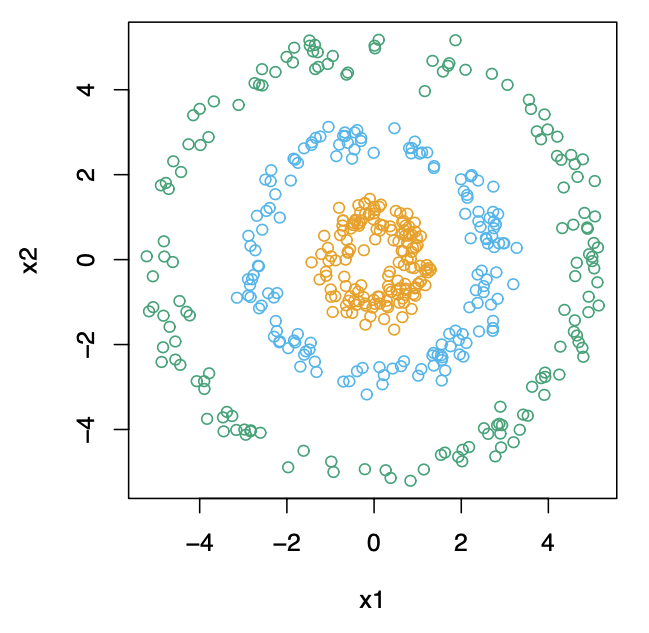Spectral clustering is a generalization of standard clustering methods, and is designed for these situations

## Clustering = graph-partition problem

Clustering can be rephrased as a graph-partition problem, with setup as below:

• a $N\times N$ matrix of pairwise similarities $s_{ii^{\prime}}\ge 0$ between all observation pairs
• represent the obeservations in an $undirected\text{ } similarity\text{ }graph$
$G=$
• $V$ : the $N$ vertices $v_i$ representing the observations
• $E$ : the edges connecting pairs of vertices if their similarity is positive, the edges are weighted by the $s_{ii^{\prime}}$

We wish to partition the graph, such that edges between different groups have low weight, and within a group have high weight. The idea of spectral clustering is to construct similarity graphs that represent the local neighborhood relationships between observations.

## Graph Laplacian

The $graph\text{ }Laplacian$ is defined by

$L=G-W$

where

• $W$ : the matrix of edge weights $W=\{w_{ii^{\prime}}\}$ from a similarity graph is called the $adjacency\text{ }matrix$
• $G$ : a diagonal matrix with diagonal elements $g_i=\sum\limits_{i^{\prime}}w_{ii^{\prime}}$, the sum of the weights of the edges connected to it. This is called $degree\text{ }matrix$

Note that this is $unnormalized\text{ }graph\text{ }Laplacian$. There are a number of normalized versions have been proposed - for example, $\tilde{L}=I-G^{-1}W$.

## Steps for spectral clustering

1. Define appropriate $similarity\text{ }graph$ (i.e., vertices and edges) that reflects local behavior of observations.

2. Get the $graph\text{ }Laplacian$ $L$ correspoding to the similarity graph $G$

3. Find the $m$ eigenvectors $Z_{N\times m}$ corresponding to the $m$ smallest eigenvalues of $L$ ($graph\text{ }Laplacian$, defined above). (For understanding, see this link and this link). This changes the data points from left to right. (See Figure 14.29 in ESL p.546)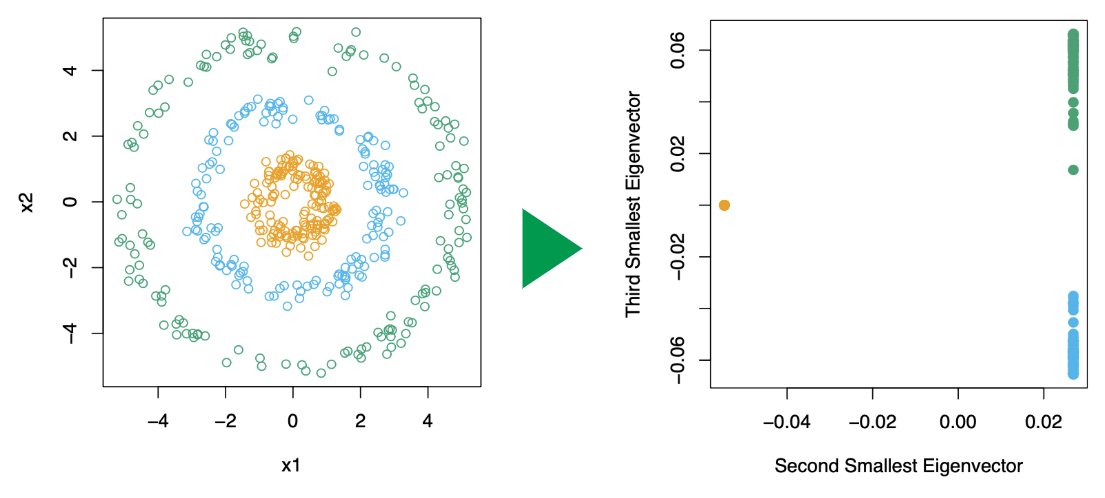4. Using a standard method like $K$-means, cluster the rows of $Z$ to yield a clustering of the original data points

# Performance evaluation

Silhouette CoefficientCalinski-Harabasz IndexDavies-Bouldin Index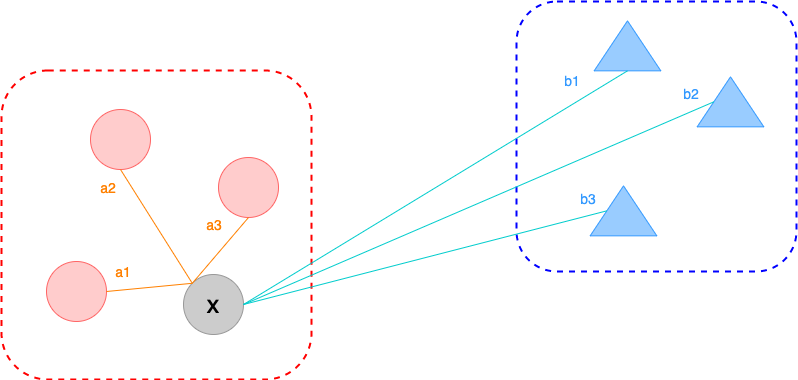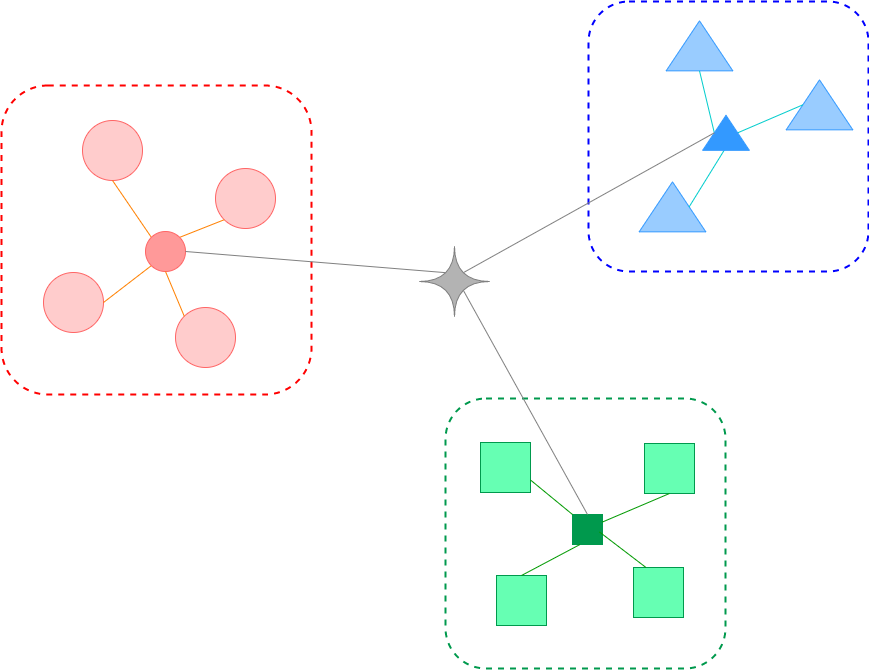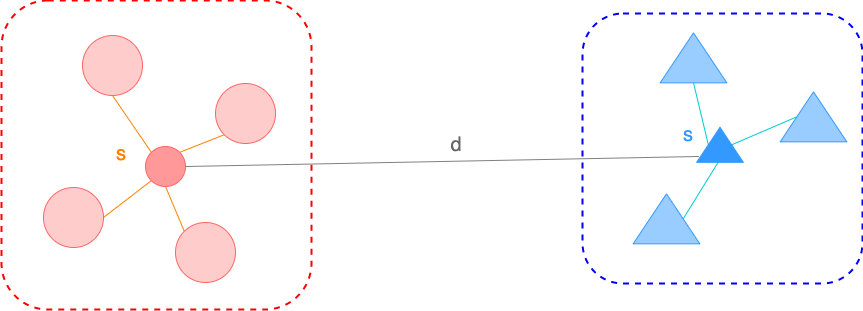Mean of scaled differences between within-cluster distance and nearest-cluster distanceThe ratio of the between-cluster dispersion and within-cluster dispersionMean of the average similarity between each cluster and its most similar(nearest) one
For a single sample, $s=\dfrac{b-a}{\max(a, b)}$
where
- $a$ : the mean distance between a sample and all other points in the same cluster
- $b$ : the mean distance between a sample and all other points in the next nearest cluster

For a set of samples, the mean of the Silhouette Coefficient for each sample
For a set of data $E$ of size $n_{E}$ which has been clustered into $k$ clusters, the Calinski-Harabasz score is defined as
$s=\dfrac{tr(B_{k})}{tr(W_k)}\times\dfrac{n_{E}-k}{k-1}$
where
- $tr(B_{k})$ : the trace of the between group dispersion matrix
- $tr(W_{k})$ : the trace of the within-cluster dispersion matrix
$W_{k}=\sum\limits_{q=1}^{K}\sum\limits_{x\in c_{q}}(x-c_{q})(x-c_{q})^{\top}$
$B_{k}=\sum\limits_{q=1}^{K}n_{q}(c_{q}-c_{E})(c_{q}-c_{E})^{\top}$
with
- $C_{q}$ : the set of points in cluster $q$
- $c_q$ : the center of cluster $q$
- $c_{E}$ : the center of $E$
The Davies-Bouldin index is defined as
$DB=\dfrac{1}{K}\sum\limits_{i=1}^{K}\max\limits_{i\ne j} R_{ij}$
where
$R_{ij}=\dfrac{s_i + s_j}{d_{ij}}$
with
- $s_i$ : the average distance between each point of cluster $i$ and the centroid of that cluster
- $d_{ij}$ : the distance between cluster centroid $i$ and $j$
• Silhouette Coefficient
• diff(within cluster distance, nearest cluster distance)
• nearest cluster distance = min(between cluster distance)
• For a given single sample point $x$,
• within cluster distance $a = mean(a1,a2,...)$
• nearest cluster distance $b = mean(b1,b2,...)$• (The Silhouette Coefficient for a set of samples)= mean(the Silhouette Coefficient for each sample)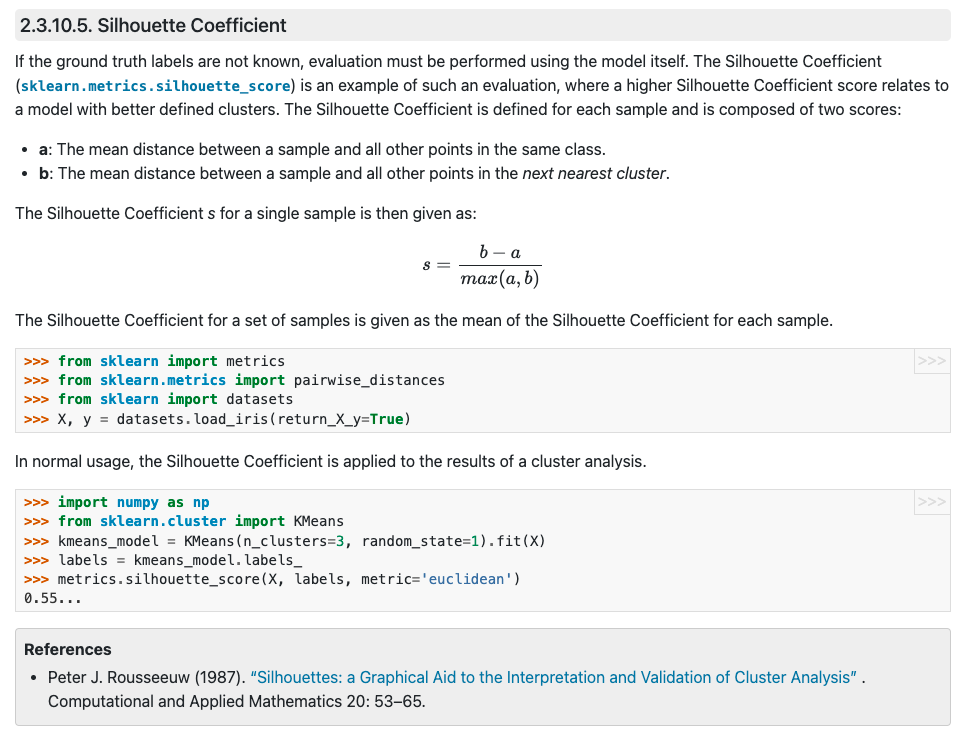• Calinski-Harabasz Index

• (Between cluster variance)/(Within cluster variance)• Davies-Bouldin Index

• mean(nearest cluster similarity)